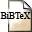# A Log-Det Inequality for Random Matrices

## Authors

M. Dörpinghaus, N. Gaffke, L. Imhof, R. Mathar,

## Abstract

We prove a new inequality for the expectation E [log det (WQ + I)], where Q is a nonnegative definite matrix and W is a diagonal random matrix with identically distributed nonneg-ative diagonal entries. A sharp lower bound is obtained by substituting Q by the diagonal matrix of its eigenvalues Γ. Conversely, if this inequality holds for all Q and Γ, then the diagonal entries of W are necessarily identically distributed. From this general result, we derive related deterministic inequalities of Muirhead- and Rado-type. We also present some applications in information theory:
We derive bounds on the capacity of parallel Gaussian fading channels with colored additive noise and bounds on the achievable rate of noncoherent Gaussian fading channels.

## BibTEX Reference Entry```@article{DoGaImMa15,
author = {Meik D{\"o}rpinghaus and Norbert Gaffke and Lorens Imhof and Rudolf Mathar},
title = "A Log-Det Inequality for Random Matrices",
pages = "1164-1179",
journal = "Siam Journal on Matrix Analysis and Applications",
volume = "36",
number = "3",
doi = 10.1137/140954647,
month = Aug,
year = 2015,
hsb = RWTH-2015-04299,
}
```Download paperDownload bibtex-file